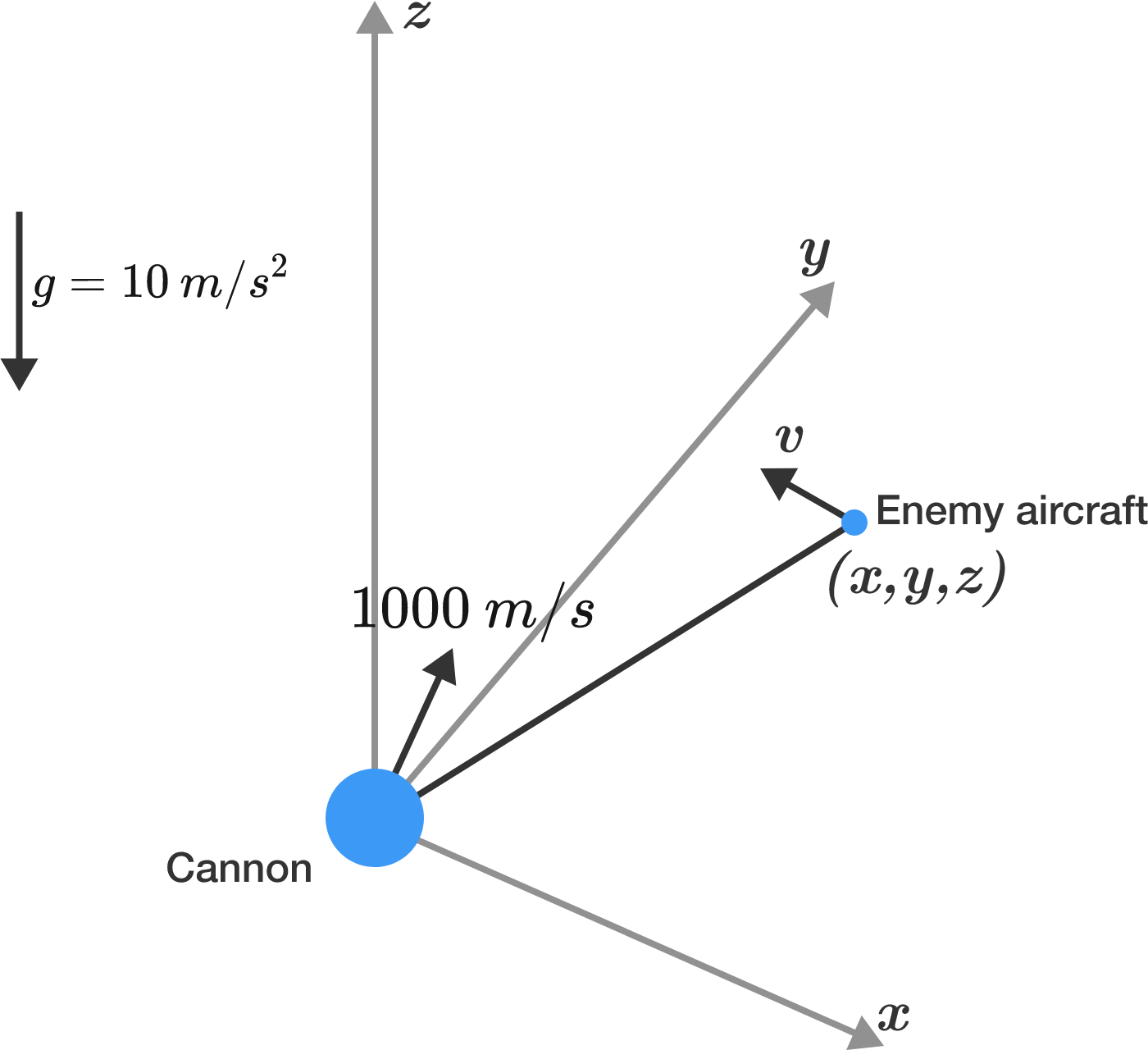# Anti-Aircraft Marksman

You are a soldier, and your base is under attack by enemy aircraft. At time $t = 0$, one such aircraft is positioned at $(x,y,z) = (1000\text{ m, }2000 \text{ m, }3000 \text{ m}).$ The aircraft has a constant velocity of $(v_x,v_y,v_z) = (-400 \text{ m/s, }+400 \text{ m/s, }+400 \text{ m/s}).$

Also at $t = 0,$ you take control of an anti-aircraft artillery cannon and fire a shot to bring down the enemy plane. The cannon has a muzzle velocity of $1000 \text{ m/s},$ and it can be aimed in any direction. Your position is $(x,y,z) = (0 \text{ m, }0 \text{ m, }0\text{ m}).$There is an ambient gravitational acceleration of $10 \text{ m/s}^2$ in the $-z$ direction (downward).

At what time (in seconds) does the artillery shell strike the enemy plane?


Details and Assumptions:

• Neglect air resistance.
• Give your answer to 2 decimal places.
• From a targeting perspective, consider the enemy plane to be a point-particle.
• You are a superlative marksman.
• If you find more than one solution, give the smaller value (provided that it's not negative).
×

Problem Loading...

Note Loading...

Set Loading...